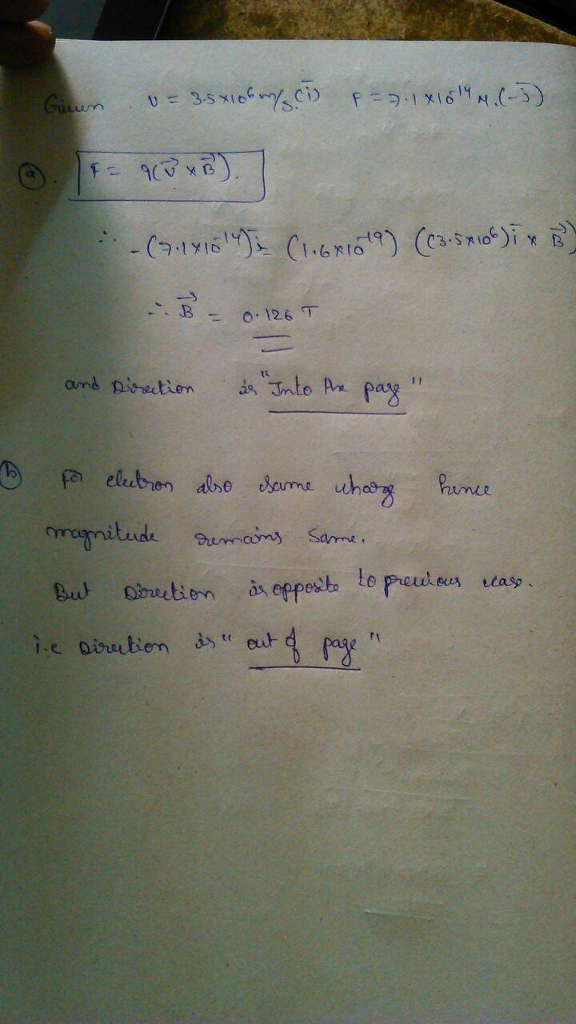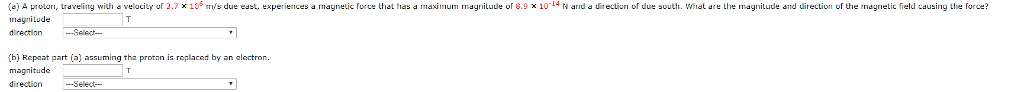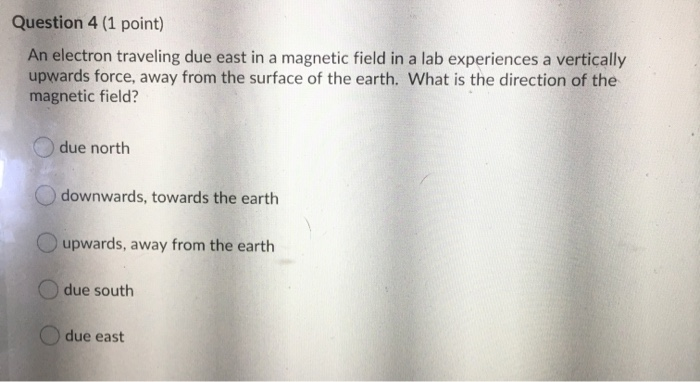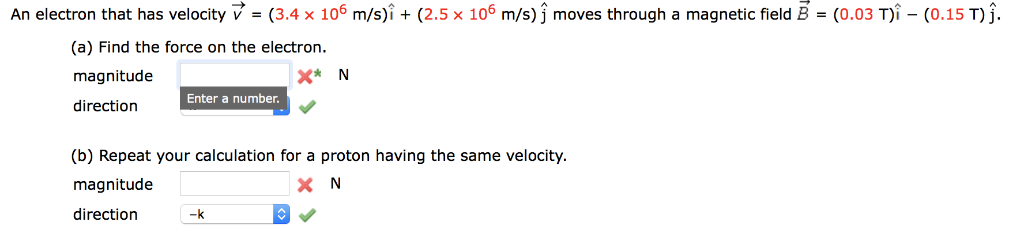# (a) A proton, traveling with a velocity of 3.5 106 m/s due east, experiences a magnetic...

(a) A proton, traveling with a velocity of 3.5 106 m/s due east, experiences a magnetic force that has a maximum magnitude of 7.1 10-14 N and a direction of due south. What are the magnitude and direction of the magnetic field causing the force?

 magnitude T

(b) Repeat part (a) assuming the proton is replaced by an electron.

magnitude T#### Earn Coin

Coins can be redeemed for fabulous gifts.

Similar Homework Help Questions
• ### a A pro on, raveling with a velocity u 3.7 x 106 m s due east,...a A pro on, raveling with a velocity u 3.7 x 106 m s due east, exper ences magnetic rce that has maxmum rnagnitude of 8.9 x 10 4 N and a dres un o due south what are the magnitude and ur ection o he md netiじfield edusny Lhe orce (b) Repaat part (a) assuming tha proton is replaced by an alectron. direction

• ### An electron traveling due east in a region that contains only a magnetic field experiences a...

An electron traveling due east in a region that contains only a magnetic field experiences a vertically downward force, toward the surface of the earth. A) What is the direction of the magnetic field? B) If it is a proton, what will be the direction of the magnetic field? Please explain the difference between electron and proton due to the current direction.

• ### Question 4 (1 point) An electron traveling due east in a magnetic field in a lab...Question 4 (1 point) An electron traveling due east in a magnetic field in a lab experiences a vertically upwards force, away from the surface of the earth. What is the direction of the magnetic field? due north downwards, towards the earth upwards, away from the earth due south due east

• ### A proton moving at 4.50 106 m/s through a magnetic field of magnitude 1.80 T experiences...

A proton moving at 4.50 106 m/s through a magnetic field of magnitude 1.80 T experiences a magnetic force of magnitude 7.80 10-13 N. What is the angle between the proton's velocity and the field? (Enter both possible answers from smallest to largest.) a. in ° b. in °

• ### A proton moving at 6.60 106 m/s through a magnetic field of magnitude 1.78 T experiences...

A proton moving at 6.60 106 m/s through a magnetic field of magnitude 1.78 T experiences a magnetic force of magnitude 7.20 10-13 N. What is the angle between the proton's velocity and the field? (Enter both possible answers from smallest to largest. Enter only positive values between 0 and 360.)

• ### A proton moving at 5.80 106 m/s through a magnetic field of magnitude 1.78 T experiences...

A proton moving at 5.80 106 m/s through a magnetic field of magnitude 1.78 T experiences a magnetic force of magnitude 8.00 10-13 N. What is the angle between the proton's velocity and the field? (Enter both possible answers from smallest to largest. Enter only positive values between 0 and 360.) _________ smaller value ° __________ larger value °

• ### A proton moving at 5.80 106 m/s through a magnetic field of magnitude 1.80 T experiences...

A proton moving at 5.80 106 m/s through a magnetic field of magnitude 1.80 T experiences a magnetic force of magnitude 8.00 10-13 N. What is the angle between the proton's velocity and the field? (Enter both possible answers from smallest to largest. Enter only positive values between 0 and 360.) smaller value ° larger value °

• ### 009 (part 1 of 3) 10.0 points A proton travels with a speed of 4.8×106 m/s...

009 (part 1 of 3) 10.0 points A proton travels with a speed of 4.8×106 m/s at an angle of 96◦ west of north. A magnetic field of 4.6 T points to the north. Find the magnitude of the magnetic force on the proton. (The magnetic force experienced by the proton in the magnetic field is proportional to the component of the proton’s velocity that is perpendicular to the magnetic field.) Answer in units of N. 010 (part 2 of...

• ### An electron that has velocity (3.4 x 10 m/s)i+ (2.5 x 106 m/s)j moves through a...An electron that has velocity (3.4 x 10 m/s)i+ (2.5 x 106 m/s)j moves through a magnetic field B (0.03 Ti (0.15 T)]. (a) Find the force on the electron magnitude X* N direction Etera mumber (b) Repeat your calculation for a proton having the same velocity magnitude direction

• ### A proton moving at 7.00 x 106 m/s through a magnetic field of magnitude 2.6 T...

A proton moving at 7.00 x 106 m/s through a magnetic field of magnitude 2.6 T experiences magnetic force of magnitude 6.9x10-13 N. What is the angle between the proton's velocity and the field? 6.2° 76.3° 13.7° 44.9°